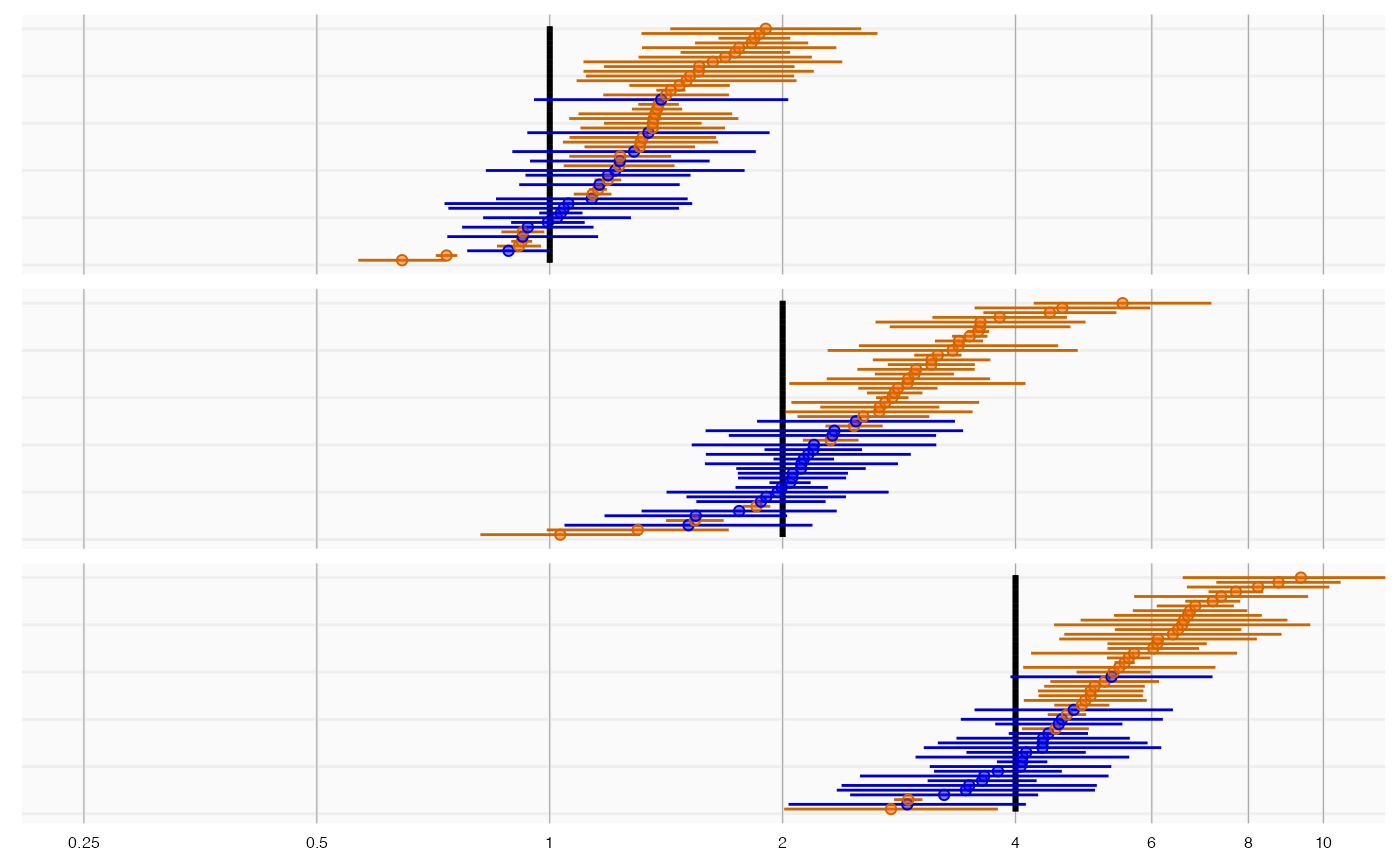Plot true and observed values, for example from a simulation study.

plotTrueAndObserved(
logRr,
seLogRr,
trueLogRr,
xLabel = "Relative risk",
title,
fileName = NULL
)

## Arguments

logRr A numeric vector of effect estimates on the log scale. The standard error of the log of the effect estimates. Hint: often the standard error = (log() - log())/qnorm(0.025). A vector of the true effect sizes. The label on the x-axis: the name of the effect estimate. Optional: the main title for the plot Name of the file where the plot should be saved, for example 'plot.png'. See the function ggsave in the ggplot2 package for supported file formats.

## Value

A Ggplot object. Use the ggsave function to save to file.

## Details

Creates a forest plot of effect size estimates (ratios). Estimates that are significantly different from the true value (alpha = 0.05) are marked in orange, others are marked in blue.

## Examples

data <- simulateControls(n = 50 * 3, mean = 0.25, sd = 0.25, trueLogRr = log(c(1, 2, 4)))
plotTrueAndObserved(data$logRr, data$seLogRr, data\$trueLogRr)